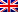# Entropy, purity and V-measure

Contenus

## Entropy, purity and V-measure

Since the full cluster (all objects of the same class are assigned to a single cluster) and the homogeneous cluster (each cluster only contains objects of the same class) are rarely reached, we aim to achieve a balance satisfactory between these two approaches. Therefore, we generally apply five well-known grouping criteria in order to evaluate the performance of the partition, which are purity, H entropy, V measure, RAND index, and F measure. first. The others are exposed on another page.

The entropy measure is used to show how sentence clusters are partitioned within each cluster, and it is known as the average of the weighted values in each cluster entropy over all clusters C = {c_1,…, c_n} :

The purity of a cluster is the fraction of the size of the cluster that represents the largest class of sentences assigned to this cluster, namely:

The overall purity is the weighted sum of the purities of the individual clusters given by:

Although purity and entropy are useful for comparing partitionings with the same number of clusters, they are unreliable when comparing partitioning with different numbers of clusters. This is because entropy and purity work on how sets of sentences are partitioned within each cluster, and this will lead to a case of homogeneity. The highest purity scores and the lowest entropy scores are usually obtained when the total number of clusters is too large, where this step will lead to being the lowest in completeness. The next measure considers both the completeness and consistency approaches.

The measurement V is known as the harmonic mean of homogeneity and completeness; i.e., V = homogeneity * completeness / (homogeneity + completeness), where homogeneity and completeness are defined as homogeneity = 1-H (C | L) / H (C) and completeness = 1-H (L | C) / H (L) where:FRFRENRUES